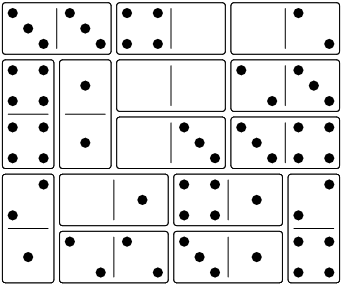# Experiments in Progress

These are new puzzles that aren’t finished yet. You can try them out and let me know what you think.

• Adding Donimoes is a puzzle I designed where you add dominoes in the given order. (1 player, double-six dominoes)

The idea was to avoid the slow setup phase at the start of the other puzzles.

### Goal

The goal is to add all the dominoes from the queue onto the board. Each problem shows the queue of dominoes to add, from left to right.

### Start

Take the two dominoes from the left end of the queue and place them on the board in the same position relative to each other.

For example, if this is the queue: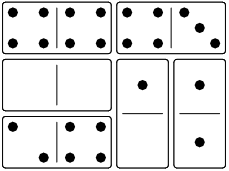Then the start position is like this: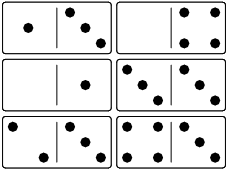Not like this: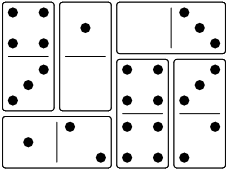### Moves

There are only two ways a domino can move.

The next domino from the queue can be added to the board if it matches at least two of the adjacent numbers on neighbouring dominoes. Those two adjacent numbers can match the two ends of the domino, or both match one end.

In this example, the 13 can be added, because it matches the 1 below and the 3 below.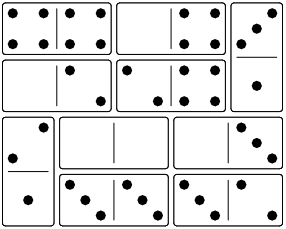In this example, the 52 can be added, because it matches the 5 beside and the 5 above. The 52 could also be added in the vertical position.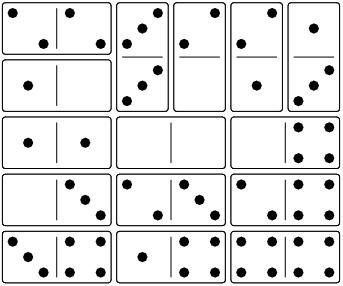#### Sliding

Move a domino one space along its long axis so that it ends up with at least one of its numbers next to an adjacent number that adds up to six, or it matches at least two of the adjacent numbers on neighbouring dominoes.

In this example, the left domino can move down, because the 1 and the 5 add to six.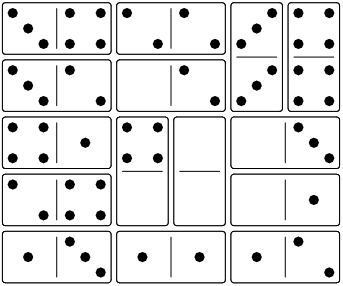The left domino can move back up, because the 1 matches the 1 above, and the 5 matches the 5 to the right.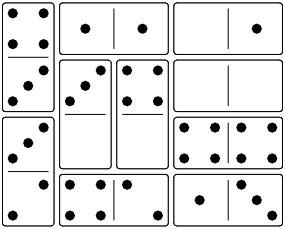#### Stay Connected

All the dominoes on the board must stay in one connected group, you can’t split the group after moving a domino.

### Problems

Here are the starting positions for several Adding Donimoes problems. The solutions are listed at the end.

#### Problem 1